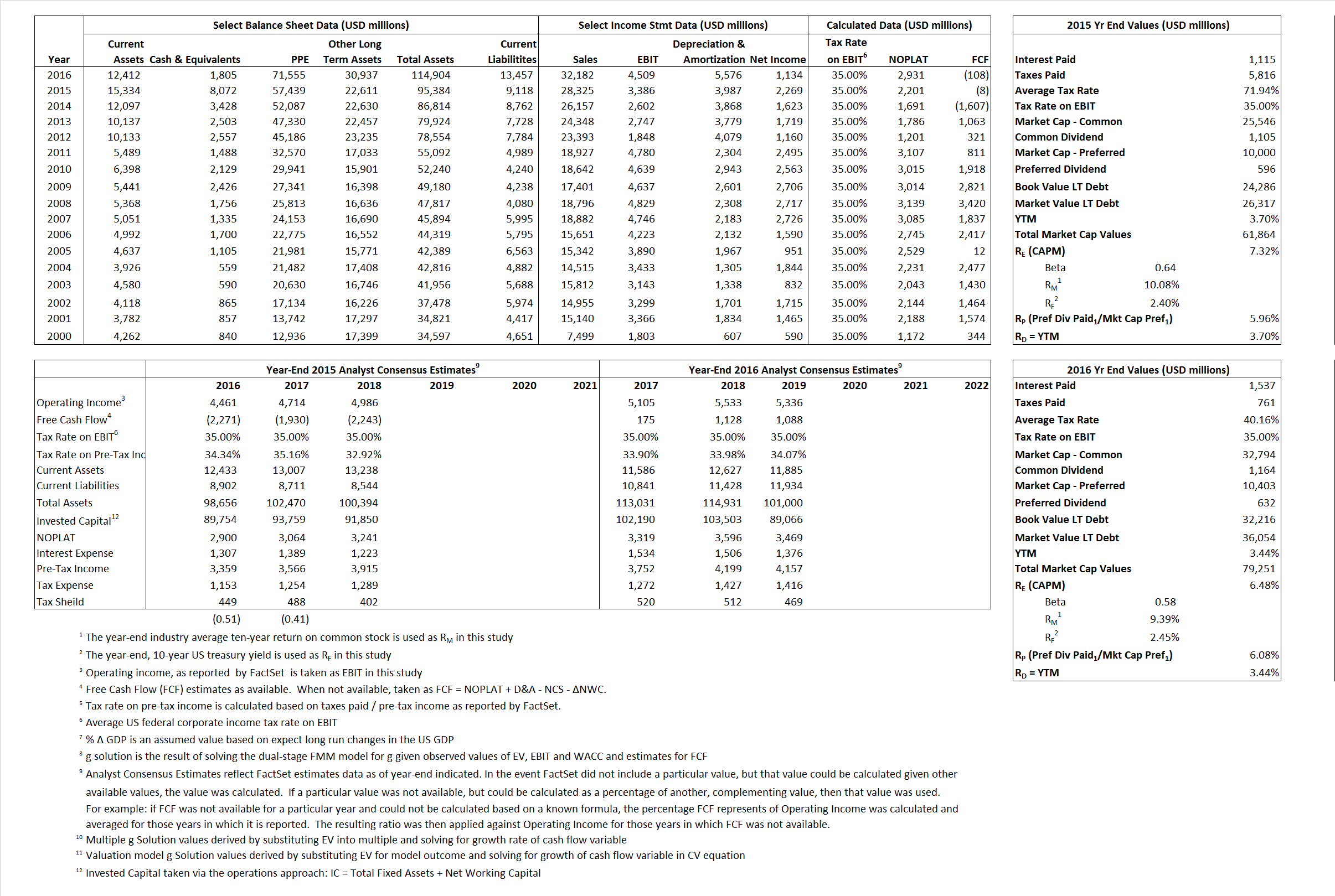# Exelon Corporation

## Analyst Listing

The following analysts provide coverage for the subject firm as of May 2016:

 Broker Analyst Analyst Email SunTrust Robinson Humphrey Ali Agha ali.agha@suntrust.com Jefferies Anthony C. Crowdell acrowdell@jefferies.com Wells Fargo Securities Neil Kalton neil.kalton@wellsfargo.com KeyBanc Capital Markets Paul T. Ridzon pridzon@key.com Guggenheim Securities Shahriar Pourreza shahriar.pourreza@guggenheimpartners.com

## Primary Input Data## Derived Input Data

### Equational Form

Net Operating Profit Less Adjusted Taxes NOPLAT  2,201  2,931$NOPLAT\, =\, EBIT\, x\, (1 \,-\, Avg \,\,Tax\,\, Rate\,\, on\,\, EBIT)$
Free Cash Flow FCF (8) (108)$FCF\,=NOPLAT\,+\,Non-Cash\,Expenses-\Delta NWC\,-\,NCS$
Tax Shield TS 802 617$TS\,=\,Interest\,\,Paid\,\,x\,\, Avg \,\,Tax\,\,Rate\,\, on\,\, Pre-Tax\,\, Income$
Invested Capital IC 86,266 101,447$IC\,=\,Fixed\,\,Operating\,\,Assets\,\,+\,\,Net\,\, Working\,\, Capital$
Return on Invested Capital ROIC 2.55% 2.89%$ROIC\,=\,\frac { NOPLAT }{ IC }$
Net Investment NetInv 12,201  20,757$NetInv\,=\,{ {IC}_{1}}-{{IC}_{0}}+Depreciation$
Investment Rate IR 554.36% 708.22%$IR\,=\,\frac {NetInv}{NOPLAT}$
Weighted Average Cost of Capital
WACCMarket 4.43%  4.41%$WACC\,=\,\frac { E }{ V } { R }_{ E }\,+\,\frac { P }{ V } { R }_{ P }\,+\,\frac { D }{ V } { R }_{ D }\left( 1- Avg\,\, Tax\,\,Rate\,\,on\,\,Pre-Tax\,\,Income \right)$
WACCBook    4.46%  3.86%
Enterprise value
EVMarket 53,792 77,446$EV\,=\,Market\,\,Cap\,\,Equity\,+\,\,Long\,\,Term\,\,Debt\,-\,Cash$
EVBook   51,760  73,608
Long-Run Growth
g = IR x ROIC
14.14%  20.46% Long-run growth rates of the income variable are used in the Continuing Value portion of the valuation models.
g = %$\Delta$ GDP    2.50%   2.50%
Margin from Operations M  11.95%  14.01%$M\,\,=\,\,\frac{EBIT}{SALES}$
Depreciation/Amortization Rate D  54.08%  55.29%$D\,\,=\,\,\frac{D+A}{EBITDA}$

## Valuation Multiple Outcomes

The outcomes presented in this study are the result of original input data, derived data, and synthesized inputs.

### model g solution

12/31/2015 12/31/2016 12/31/2015 12/31/2016 12/31/2015 12/31/2016

EV/SALES$\frac {EV}{Sales} \,= \,\frac{ROIC\, -\, g}{ROIC\,(WACC\,-\,g)}\,(1\,-\,T)\,(M)$

1.90  2.41  -0.55%  -2.03%  -4.42%  -7.23%

EV/EBITDA$\frac {EV}{EBITDA} \,= \,\frac{ROIC\, -\, g}{ROIC\,(WACC\,-\,g)}\,(1\,-\,T)\,(1\,-\,D)$

7.30 7.68   -0.55% -2.03%   -4.42%  -7.23%

EV/NOPLAT$\frac {EV}{NOPLAT} \,= \,\frac{ROIC\, -\, g}{ROIC\,(WACC\,-\,g)}$

24.44 26.42  -0.55% -2.03%   -4.42%  -7.23%

EV/FCFOPS$\frac {EV}{FCF_{OPS}} \,= \,\frac{ROIC\, -\, g}{ROIC\,(WACC\,-\,g)}\,(1\,-\,T)$

-6723.94 -717.09  -0.55% -2.03%   -4.42% -7.23%

EV/EBIT$\frac {EV}{EBIT} \,= \,\frac{ROIC\, -\, g}{ROIC\,(WACC\,-\,g)}\,(1\,-\,T)$

15.89 17.18  -0.55% -2.03%   -4.42% -7.23%

EV/IC$\frac {EV}{IC} \,= \,\frac{ROIC\, -\, g}{WACC\,-\,g}$

.62  .76  -0.55% -2.03%   -4.42% -7.23%